# Tech Tips

### Type and Characteristics of Amplifier

If you search for "amplifier" on the Internet, you will get results for many web sites about audio power amplifier. But the "amplifier" itself is not just for audio. The device that amplifies something is called an amplifier. Of course, in the electrical circuit, including audio power amplifier, the various signals are exchanged as electrical signals. So, the amplifier is for amplifying the signal flowing in the electric circuit, and it amplifies the input current or voltage. It operates amplifying the electric signal output from the various sensor and making it easy to perform analog-to-digital conversion.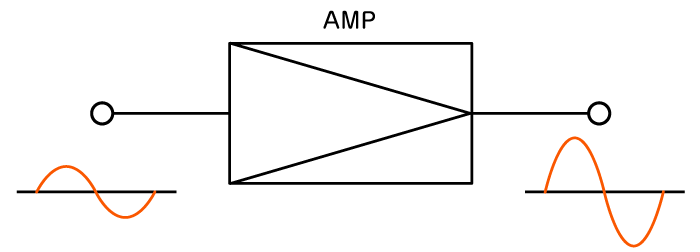An amplifier is a representative of analog circuits, and it is so central that there is nothing if the amplifiers are removed from the analog circuits. In addition, since the amplifier can supply any electric current and voltage (or electric power), it can also be used as a power supply simulator. In fact, the power supply is also a kind of amplifier. DC power supplies are also called unipolar (power supply) because of being able to supply positive charge only.

We call a two-quadrant bipolar power supply that can supply current sources of positive and negative charge, and a four-quadrant bipolar power supply that can supply also current sink. We will introduce the four-quadrant bipolar power supply in detail at the last chapter.

There are two main types of amplifiers called "linear amplifier" and "digital amplifier".
A "linear amplifier" has a frequency domain where the output signal is linearly amplified with respect to the input signal and a domain where it is nonlinear due to the characteristics of the amplifying elements such as transistors and FETs that make up the electric circuit. In particular, the output signal becomes non-linear in the region near zero, the ON/OFF operation of input signal to the element causes distortion in the output waveform. Therefore, it becomes important in which region the input signal is used, and it falls into three categories, Class-A, Class-B and Class-AB (including Class-AB1 and Class-AB2).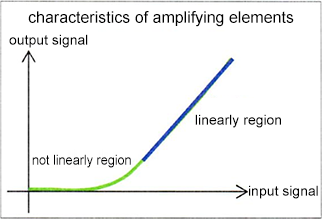#### Class-A amplifiers

This amplifier uses only the linearly amplified region of the element. Therefore, although the linearity is high, it is necessary to supply a bias current (or voltage) even when the input signal is close to zero, which is disadvantageous in that the efficiency is degraded and the heat generation is large. That is, to ensure that the output signal is correct, it always keeps a constant bias current even when the input signal is zero.

#### Class-B amplifiers

This amplifier uses both the non-linearly and the linearly amplified region of the element as it is. Therefore, when the input signal is near zero, the output signal is also zero and distortion occurs. Instead, there is no need for bias current like Class-A amplifiers, and the efficiency is improved.

#### Class-AB amplifiers

It is an amplifier that has a good reputation between Class-A and Class-B. Distortion is canceled by adding a bias current to a Class-B amplifier.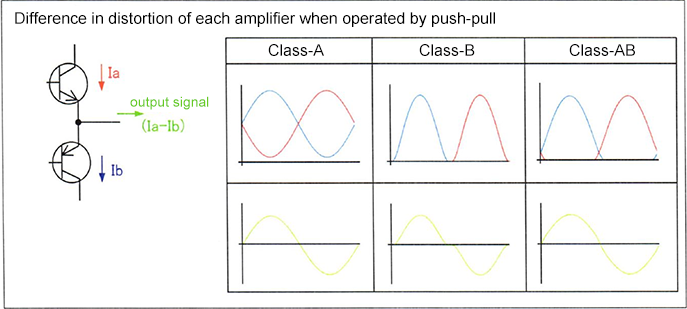Another amplifier is a "digital amplifier" also called switching amplifier, class-D amplifier. This is more efficient and smaller than linear amplifiers by using switching technique such as PWM. It is mainly used for compact audio power amplifiers such as automotive applications. Although MOSFETs and IGBTs are used as switching devices, there is also the problem that the frequency band of the corresponding input signal is narrow.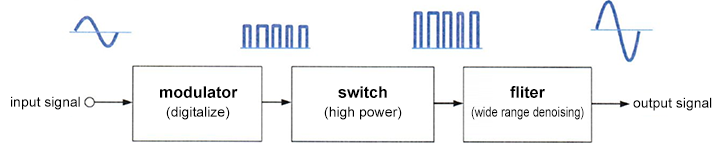### Conditions Necessary for Stable Operation of the Amplifier

So far, we have explained the type and characteristics of the amplifier. From here, we will introduce what kind of things we should be careful about when designing and implementing amplifier.

#### Frequency band

In order to the electric current and voltage output values to stabilize, it is necessary to understand factors that inhibit them. The first factor is the frequency band. The frequency band corresponds to the operating speed of the amplifier. At high frequencies, the amplifier cannot keep up with the input signal and the amplitude of signal decreases. The figure shows the frequency until the amplitude reaches -3 dB in the frequency band.For example, when the frequency band of a 120 V rated amplifier is 20 kHz, even if it tries to output a ± 20 V sine wave at 20 kHz, the output amplitude becomes 70% at -3 dB, so it becomes a ± 14 V sine wave. Therefore, it is necessary to select an amplifier with a frequency band with a margin for the frequency you want to use. The rise time and fall time are related to the frequency band. Generally, the rise time of the response speed (= frequency band) fc (Hz) amplifier can be obtained by tr ≑ 0.35/fc.

#### Slew rate

The second factor is a slew rate that represents the response speed of the amplifier. This shows the maximum voltage rise rate of the amplifier. Generally, it is expressed by the amount of voltage change per micro second. The response speed of the amplifier may be limited by the frequency band or by this slew rate. When the step response is limited by the slew rate, the rising waveform becomes straight as shown in the figure.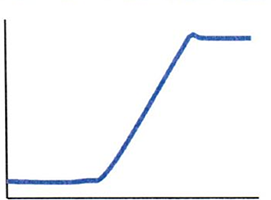the case limited by slew rate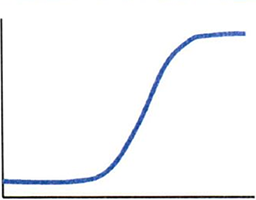the case limited by frequency band

#### Inductive load

So far, it was due to speed, but now we will introduce things related to load. The first factor is inductive load.
In the case of inductive load, the voltage-current relationship is V = L times di/dt with respect to the inductance value L, and the voltage generated when trying to operate at high speed under constant-current (CC) control raises problems.

For example, when trying to output a square wave with a fast rise speed, the desired waveform may not be obtained because the voltage is limited by the overvoltage protection. In such case, it is necessary to slow the rising speed of the input signal and select a model that supports the generated voltage.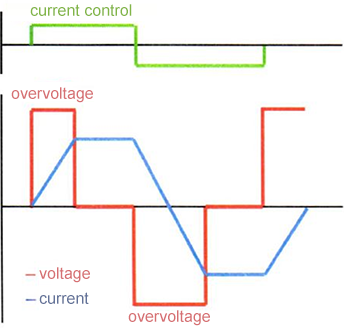In addition, using a step-like signal such as digital control for the input signal will generate many voltage pulses as well. As these pulses may create a problem, it is recommended to use continuous waveform input signal as much as possible.

On the other hand, overvoltage protection also limits the output signal. However, if the output signal is suddenly turned off, the protection does not work and a large voltage may be generated from the inductive load.

#### Capacity load

The second factor is capacity load. For capacitive load, the voltage-current relationship is I = C times dV/dt for capacitance C. Contrary to inductive load, large current is required when trying to operate at high speed under constant-voltage (CV) control. When handling a large capacity, understand the load characteristics and the output characteristics of the power supply before use.

#### Diode load

The third factor is a diode load. Under constant-current (CC) control, even if the current control is zero under no load condition, the output voltage rises to the positive or negative overvoltage protection level under the influence of a slight offset. This means that a diode or other load that only allows forward current can output signal an excessive voltage in the reverse direction even when the current control is zero. If this exceeds the withstand voltage of the load, it may cause a failure, so it is necessary to take measures such as inserting a protective diode in the reverse direction.

#### Capacitance and inductance of cable

Last factor is the cable. When operating the amplifier at high speed, we cannot ignore the effects of the capacitance and inductance of the cable for output signal. In high-voltage amplifiers, the cable has a capacitance between the output wire and the shield, so the capacitance affects the rising speed of the voltage waveform. The longer cable has the greater capacity. This is the reason for using a low electrical resistance cable among music enthusiasts and building a system that minimizes the length of cable.

In addition, in the low-voltage, high-current model, the inductance of the cable and the inductance generated by the wiring method greatly affect the rise speed of the current waveform. This can be mitigated to some extent by making the current loop smaller, such as twisting the wiring.

### Four-quadrant Bipolar Power Supply

Finally, let us introduce the four-quadrant bipolar power supply, which is a high-performance amplifier, as an evolution of the amplifier. The amplifier basically has an output current sink. As a result, constant-voltage operation is possible even with capacity loads, inductive loads and their combined loads. Moreover, because it responds quickly, it can be said that it is an ideal power supply. A general power supply can only output electric current in one direction. But a four-quadrant bipolar power supply can output voltage in both positive and negative directions.

Moreover, it has current sink and source function. When applying alternating current to inductive load or capacity load, the same voltage may have positive and negative currents. A four-quadrant bipolar power supply is required to drive such a load.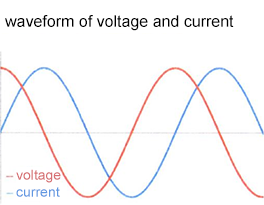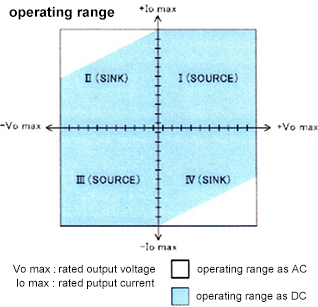Under constant-voltage (CV) control, a four-quadrant bipolar power supply output voltage corresponding to the input signal. At this time, the output current can take value freely if it is within the rating. Similarly, under constant-current (CC) control, it outputs current according to the input signal. At this time, if the output voltage is within the rating, it can be a positive or negative free value.

However, because the output protection is performed by the overvoltage protection and the over current protection, the desired waveform may not be obtained. It is desirable to operate so that both voltage and current are within the rating, and it is important for stable use of the power supply to understand the characteristics of the load.